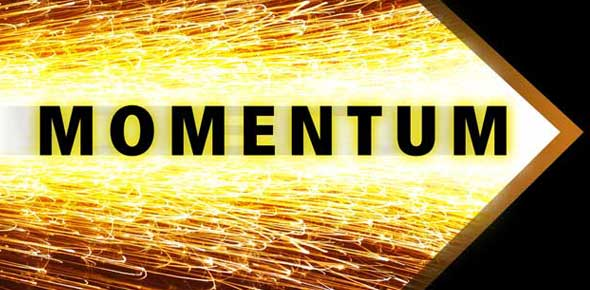# Ch 11.4 Forces Transfer Momentum

12 Questions | Total Attempts: 244SettingsComplete this activity with your shoulder partner.

• 1.
(368)  Write the definition for momentum
• 2.
(369) Write out the formula for momentum include also the units used for momentum.
• 3.
How does an object's mass and velocity affect its momentum?
• 4.
A 3Kg ball is moving with a velocity of 1m/s.  What is the ball's momentum?  _____kg m/s
• 5.
What is the momentum of a 0.5kg ball moving 0.5 m/s?  ____kg m/s
• 6.
Write the definition for collision.
• 7.
How can collision affect the momentum of an object?
• 8.
Write down what is meant by "conservation of momentum"?
• 9.
(372)  Under what condition is momentum not conserved?
• 10.
(373)  A car and a truck are traveling at the same speed.  Which has more momentum?
• 11.
One football player in motion hits another at rest.  Describe what happens to their momentum.
• 12.
A 50 kg person is running at 2m/s.  The persons resulting momentum is _____kg m/s.
Related TopicsBack to top